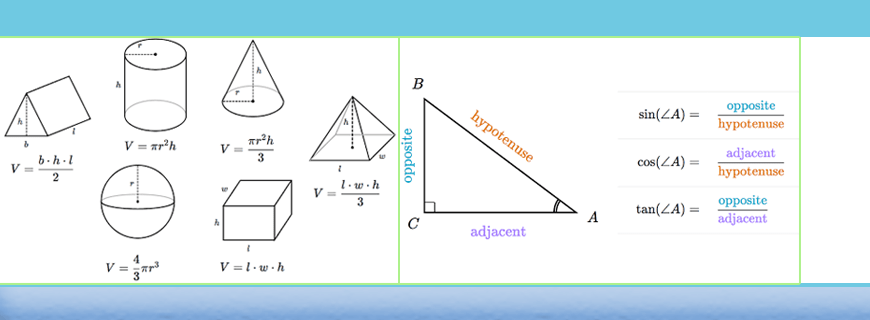# Geometry Assignments HelpGet a Free Quote
It's free and always will be.
Page - +
words
NWL

## Geometry Assignments Help

Geometry is an essential part of mathematics, particularly for the high school and college students. An understanding of the features and relationships of diverse geometric objects can be in various practical contexts-interpreting a diagram, assessing the quantity of wood needed to frame a roof, rendering computer graphics, or scheming a sewing pattern for the most efficient use of a cloth, etc. Though there are many types of geometry students learn throughout their career, school mathematics is mostly devoted to plane Euclidean geometry, studied both synthetically and analytically, i.e. with coordinate and without coordinate. In colleges, students learn other forms of geometry which is called mensuration and their practical applications. In any standard, assignments on Geometry is an obvious part of a course curriculum. Students are given various critical problems on Geometry through these assignments, which sometimes seem too critical to be solved and submitted within the given respective deadlines. In such circumstances, students take the help of Geometry Assignment Help provided by BookMyessay.

### Overview of Geometry at Higher Levels

Here are some important aspects of Geometry that the high school students frequently observe –

• Congruence
In geometry, problems are often given to establish congruence of two or more geometric figures. There are some theorems frequently used to prove congruence between two geometrical figures, which the students use while solving related problems.
• Similarity of triangles, Right Triangles, and Trigonometry –
In this categories students learn to handle following types of geometrical problems –

1. Understand similarity in terms of similarity transformations
2. Prove theorems involving similarity
3. Define trigonometric ratios and solve problems involving right triangles
4. Apply trigonometry to general triangles
• Circles – This part of geometry involves the following –
1. Understand and apply theorems about circles
2. Find arc lengths and areas of sectors of circles
• Using equations to express Geometric Properties
1. Interpreting the geometric description and the equation for a conic section
2. Application of coordinates to prove geometric theorems algebraically
• Different patterns of Geometric measurements and dimensions
1.  Explain volume formulas and use them to solve problems
2. Finding relations between two-dimensional and three-dimensional Geometrical objects
• Modeling with Geometry
1. Selecting and applying Geometric concepts in modeling

Trigonometry is the most vital part of advanced Geometry. The definitions and applications of sine, cosine, and tangent are the basis of many practical world problems involving applied mathematics. These are the parts of Pythagorean Theorem and fundamental in many practical and theoretical situations. The Pythagorean Theorem is further associated with the non-right angled triangles by the Law of Cosines.

Analytic geometry, on the other hand, links algebra with geometry, resulting in the prevailing systems of analysis and problem solving. As a number line shows numbers with positions in one dimension, a pair of perpendicular axes shows pairs of numbers with locations in two dimensions.

So, students learn the systems, theorems and procedures to solve problems that relate various above mentioned Geometrical aspects. At the higher levels the types of Geometry changes and students learn differential geometry, mensuration and topology more intensively.

Undoubtedly, it is a dynamic subject that provides students with experimental and modeling tools. These tools help the students to examine interesting geometric phenomena in much the similar way as the various algebraic systems allow them to test various algebraic phenomena.

### Help with Geometry Assignment Writing

Geometric assignments are the toughest assignments in mathematics. Because, at higher standards, it involves analysis of figure, preparation of equation and then a solution of the equation or getting into a conclusion. Assignments look tougher when those involve any practical issue like measurement of height of a monument or mountain peak or volume of a reservoir, etc. Assignments are also given on three dimensional figures like circle, ellipse, hyperbola and many more. Naturally, students look for professional help with Geometry assignment writing from experienced teachers, who can make the job easier, faster and accurate for the students. BookMyEssay has appointed highly qualified individuals who have years of experience in solving a wide array of mathematical assignment problems including geometrical problems. As a result, over the years, thousands of students, right from school levels to graduation and post-graduation levels are immensely benefited through Geometry Assignment help service provided by BookMyEssay.

### Moreover, These Assignment Writers Ensure –

• 100% plagiarism free writing.
• Complete adherence to the guidelines provided by the teacher or lecturer.
• Timely delivery of the assignments.

### Features of BookMyEssay

Some mention worthy features of BookMyEssay are as follows –

• Students help desk remains open for 24/7.
• Students can ask for amendments and rectifications in the completed assignments as many times as required.
• Whenever required, students can ask for emergency academic help service when a writer assists a student to complete an assignment with a few hours.
• The service is quite affordable.
• Payment system is very easy and safe.

We always deliver the best quality assignment and Geometry homework help service to the students of mathematics. After getting the relevant help from these professional geometry assignment writers, students always achieve the best grades in their assignments.